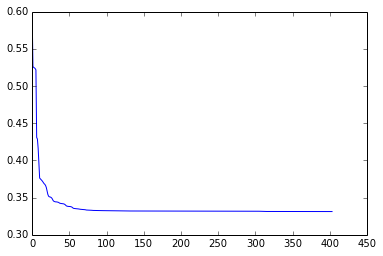# Using Mirador for Statistical Modeling¶

Mirador is a software tool for exploratory analysis of complex datasets. It has been developed as a collaboration between Fathom Information Design and the Sabeti Lab at Harvard University. This notebook goes over the use of Mirador as the first step in statistical modeling. Our goal is train a logistic regression and a neural network predictors using the explanatory variables that we find with Mirador. We will use the Hepatitis dataset that is included in the examples folder.

## Finding explanatory variables¶

After opening the Hepatitis dataset, Mirador should look something like this:From the main interface, you can inspect the plots between any pair of variables and set ranges dynamically and see the effect on the plots. Here, we are interested in finding variables we can use to model a predictive model for Hepatitis outcome. In order to find these variable, we can rank all the variables in the dataset by their correlation with the OUTCOME variable. If we hover the mouse over the variable name in the row corresponding to OUTCOME, we will se the title changing to "Sort OUTCOME". If we now click the title, the columns will be re-arranged in decreasing order of correlation strength with OUTCOME from left to right:If we open the profile view, by clicking the profile icon inside the OUTCOME variable, we will see a graph representing all the variables that are associated with OUTCOME at the current P-value, in the order that resulted from the sorting we just did. We can hover the dots to reveal the name of the variables, and clicking on them will take us back to the plot between the selected variable and outcome in the main screen. By inspecting this profile, we can start deciding which variables could be included as explanatory factors for OUTCOME, and select a range of contiguous variables using the tooltips right underneath the profile:(Tip: we cannot remove variables directly from the profile, but what we can do is to can click on them in the profile, close the corresponding column in the main view, and then return to the profile and do the selection.)

One we have defined our set of explanatory variables, we can use the "Export selection" button to save the data for these variables. The currently set ranges will also be applied in the export, e.g.: if the range AGE variable is set as 20-50, only the patients within that age range will be included in the export. Mirador will not only save the data, but also the variable names, types, and configuration (P-value, etc.)

## Imputing missing values¶

A typical problem in any kind of datasets, but particularly so with survey, health data, is the presence of missing values due to non-response, selective testing, etc. We can simply ignore the rows that contains missing values, but this decreases the amount of data we can work with, weakining any subsequent statistical analysis. Here we will use the Amelia package for R to impute missing values in our exported data, as a preliminary step before model learning. In the following R code, we will load the data we exported from Mirador, and generate 5 imputed datasets. Amelia allows you to inspect the quality of the imputation for the different variables, generate a map of the missing values, among other things.

In :
# We load the rpy2 so we can run R code in the notebook
%load_ext rpy2.ipython

# Load Amelia library and the data
%R library(Amelia)
%R hep <- read.table('profile-data.tsv', sep = "\t", header=TRUE, na.strings="?")

# Define list of nominal (categorical) variables
%R nom_vars = c("OUTCOME", "VARICES", "FATIGUE", "SPIDERS", "ASCITES", "MALAISE", "HISTOLOGY")

# Generate 5 imputed data frames
%R imputed <- amelia(hep, m = 5, noms = nom_vars)

# Missingness map
%R missmap(imputed)

# Compare observed density with imputed density
%R compare.density(imputed, var = "PROTIME")
%R compare.density(imputed, var = "ALBUMIN")
%R compare.density(imputed, var = "ASCITES")

# "Quality" of imputation (cannot be generated for nominal variables)
%R overimpute(imputed, var = "PROTIME")
%R overimpute(imputed, var = "ALBUMIN")

# Write out the 5 imputed data frames
%R write.amelia(obj=imputed, file.stem="imputed-data", format="csv", row.names = FALSE)

Loading required package: foreign
Loading required package: Rcpp
Loading required package: RcppArmadillo
##
## Amelia II: Multiple Imputation
## (Version 1.7.2, built: 2013-04-03)
## Copyright (C) 2005-2014 James Honaker, Gary King and Matthew Blackwell
## Refer to http://gking.harvard.edu/amelia/ for more information
##

-- Imputation 1 --

1  2  3  4  5  6  7  8  9 10 11 12 13 14 15

-- Imputation 2 --

1  2  3  4  5  6  7  8  9

-- Imputation 3 --

1  2  3  4  5  6  7  8  9

-- Imputation 4 --

1  2  3  4  5  6  7  8  9 10 11 12 13 14 15

-- Imputation 5 --

1  2  3  4  5  6  7  8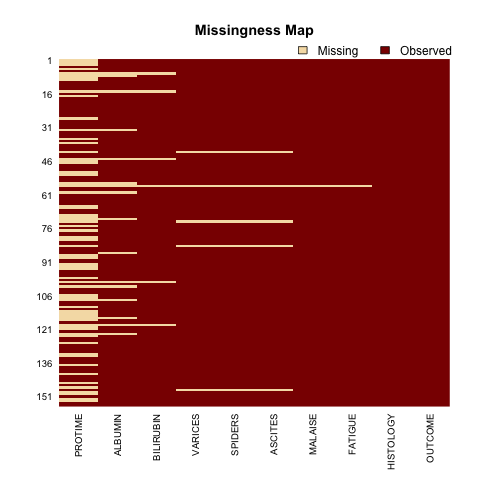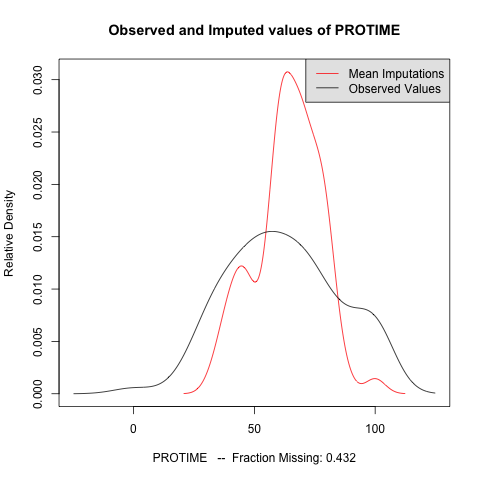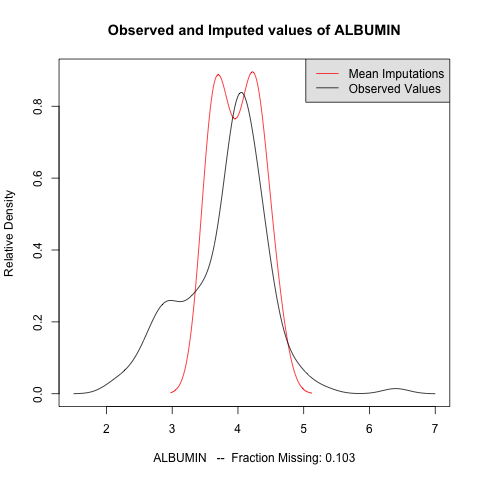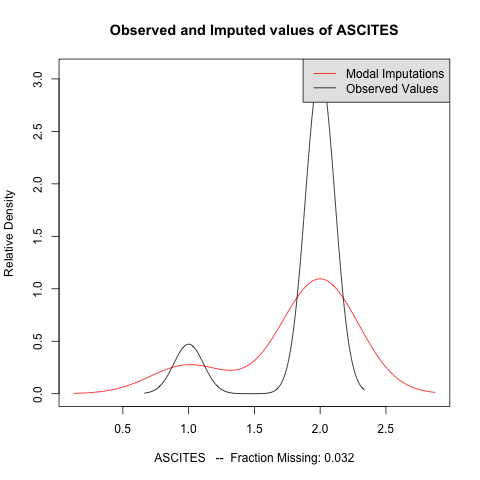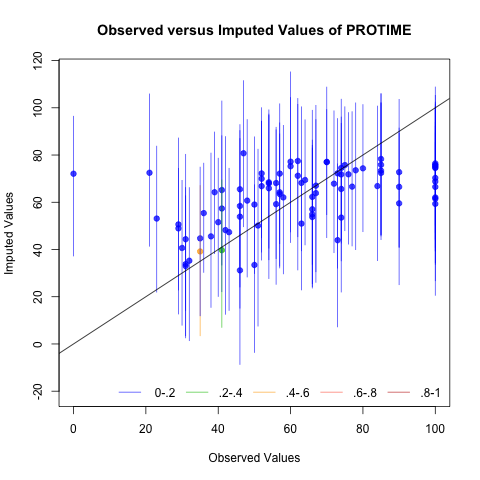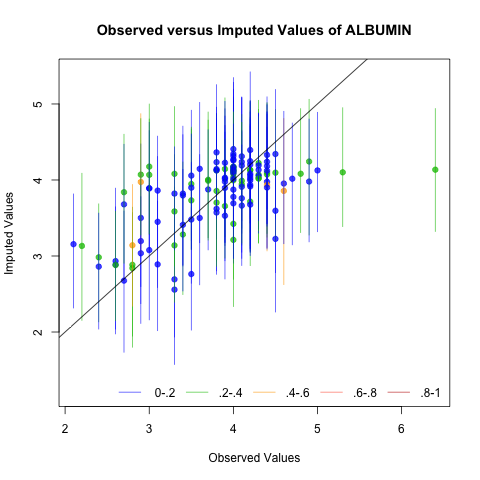## Learning models from data¶

We will now use our imputed data to train models that can predict outcome for new patients, given the information only on the variables we chose as explanatory. We implemented two machine learning algorithms, Logistic Regression and Neural Network, based on the notes from the Machine Learning course from Andrew Ng (some notes about the LR and NN sections of the Coursera class). Both algorithms the imputed data and a few parameters, split the data into two sets, 70% for training and 30% for testing, and output the results and a plot showing the progress of the training procedure.

However, we first need to normalize the categorical values in our data, so they are either 0 or 1 to be properly handled by the learning algorithms. The following code will take the first imputed dataset, and normalize the values for the variables of type category. The types of the exported variables are stored in a dictionary file that Mirador exported alongside with the data:

In :
import csv, sys

input_file = "imputed-data1.csv"
dict_file = "profile-dictionary.tsv"
norm_file = "learn-data.csv"

ifile = open(input_file, 'rb')
dfile = open(dict_file, 'rb')
ofile  = open(norm_file, 'wb')

types = []
try:
dict = csv.reader(dfile, delimiter='\t')
for row in dict:
types.append(row)
finally:
dfile.close()

try:
reader = csv.reader(ifile)
writer = csv.writer(ofile, delimiter=',', quotechar='"', quoting=csv.QUOTE_MINIMAL)
titles = reader.next()
writer.writerow(titles)
for row in reader:
nrow = [''] * len(row)
for i in range(0, len(row)):
if types[i] ==  'category':
nrow[i] = '0' if row[i] == '1' else '1'
else:
nrow[i] = row[i]
writer.writerow(nrow)
finally:
ifile.close()
ofile.close()

In :
# To embed the plots inside the notebook
%matplotlib inline


### Logistic Regression¶

In logistic regression, the assumption is that the classification of the output variable (in this case, either the patient recovers or not) can be made by constructing a line (the decision boundary) separating the areas in the parameter space (the explanatory variables) that correspond to each possible output. If the decision boundary is not linear, then the logistic regression won't give a good predictive model.

In :
# Parameters for the LR algorithm, run this cell before running the predictor!

filename = "learn-data.csv"

alpha = 0.001     # Learning coefficient (ignored when using BFGS minimization)
gamma = 0.08      # Regularization coefficient

threshold = 1E-5  # Default convergence threshold

showp = True      # Show minimization plot
gcheck = False    # Gradient check
useBfgs = True    # Use BFGS algorithm for minimization of cost function
testMode = False  # Test mode: runs the algorithm testCount times
testCount = 1     # Number of test runs

In :
# This code implements a logistic regression predictor. The dependent variable
# must be binary and the first column in the data frame, and all the independent
# categorical variables must be binary as well.
# Requires:
# pandas http://pandas.pydata.org/
# numpy http://www.numpy.org/

import sys
import pandas as pd
import numpy as np
from scipy.optimize import fmin_bfgs
import matplotlib.pyplot as plt

def sigmoid(v):
return 1 / (1 + np.exp(-v))

def cost(theta, X, y, gamma):
h = sigmoid(np.dot(X, theta))
terms =  -y * np.log(h) - (1-y) * np.log(1-h)

prod = theta * theta
prod = 0
penalty = (gamma / (2 * M)) * np.sum(prod)

return terms.mean() + penalty

def gradient(theta, X, y, gamma):
M = X.shape
N = X.shape

# Note the vectorized operations using numpy:
# X is a MxN array, and theta a Nx1 array,
# so np.dot(X, theta) gives a Mx1 array, which
# in turn is used by the sigmoid function to
# perform the calculation component-wise and
# return another Mx1 array
h = sigmoid(np.dot(X, theta))
err = h - y
# err is a Mx1 array, so that its dot product
# with the MxN array X gives a Nx1 array, which
# in this case it is exactly the gradient!
costGrad = np.dot(err, X) / M

regCost = (gamma / M) * np.copy(theta)
regCost = 0

grad = costGrad + regCost

global gcheck
if gcheck:
ok = True
epsilon = 1E-5
maxerr = 0.01
grad1 = np.zeros(N);
for i in range(0, N):
theta0 = np.copy(theta)
theta1 = np.copy(theta)

theta0[i] = theta0[i] - epsilon
theta1[i] = theta1[i] + epsilon

c0 = cost(theta0, X, y, gamma)
c1 = cost(theta1, X, y, gamma)
grad1[i] = (c1 - c0) / (2 * epsilon)
diff = abs(grad1[i] - grad[i])
if maxerr < diff:
print "Numerical and analytical gradients differ by",diff,"at argument",i,"/",N
ok = False
if ok:
print "Numerical and analytical gradients coincide within the given precision of",maxerr

return grad

def debug(theta):
global params
global values
(X, y, gamma) = params
value = cost(theta, X, y, gamma);
values = np.append(values, [value])
# print value

def train(params, alpha, threshold, useBfgs):
global values
(X, y, gamma) = params
M = X.shape
N = X.shape

if useBfgs:
print ''
print 'Running BFGS minimization...'
theta0 = np.ones(N)
thetaOpt = fmin_bfgs(cost, theta0, fprime=gradient, args=params, gtol=threshold, callback=debug)
return [True, thetaOpt]
else:
print ''
print 'Running simple gradient descent...'
theta = np.ones(N)
count = 0
cost0 = cost(theta, X, y, gamma)
conv = False
while not conv:
grad = gradient(theta, X, y, gamma)
theta = theta - alpha * grad

cost1 = cost(theta, X, y, gamma)
diff = cost1 - cost0
cost0 = cost1
count = count + 1
#print count, cost1, diff
values = np.append(values, [cost1])
if 3 < diff: break
conv = np.linalg.norm(grad) < threshold
return [conv, theta]

# Main -----------------------------------------------------------------------------

# Loading data frame and initalizing dimensions
df = pd.read_csv(filename, delimiter=',', na_values="\\N")
M = df.shape
N = df.shape
print 'Number of independent variables:', N-1
print 'Number of data samples         :', M

y = df.values[:,0]

# Building the (normalized) design matrix
X = np.ones((M, N))
for j in range(1, N):
# Computing i-th column. The pandas dataframe
# contains all the values as numpy arrays that
# can be handled individually:
values = df.values[:, j]
minv = values.min()
maxv = values.max()
X[:, j] = (values - minv) / (maxv - minv)

rates = np.array([])
for iter in range(0, testCount):
if testMode: print "-------> Iteration test",iter

# Create training set by randomly choosing 70% of rows from each output
# category
i0 = np.where(y == 0)
i1 = np.where(y == 1)
ri0 = np.random.choice(i0, size=0.7*i0.shape, replace=False)
ri1 = np.random.choice(i1, size=0.7*i1.shape, replace=False)
itrain = np.concatenate((ri1, ri0))
itrain.sort()

Xtrain = X[itrain,:]
ytrain = y[itrain]

values = np.array([])
params = (Xtrain, ytrain, gamma)
[conv, theta] = train(params, alpha, threshold, useBfgs)

if conv:
print 'Convergence!'
print '{:10s} {:2.4f}'.format('Intercept', theta)
names = df.columns.values[1: N]
for i in range(1, N):
print '{:10s} {:3.5f}'.format(names[i-1], theta[i])
else:
print 'Error: cost function increased...'
print 'Try adjusting the learning or the regularization coefficients'

# Calculating the prediction rate by applying the trained model on the remaining
# 30% of the data (the test set), and comparing with random selection
ntot = 0
nhit = 0
nran = 0
for i in range(0, M):
if not i in itrain:
ntot = ntot + 1
p = sigmoid(np.dot(X[i,:], theta))
if (y[i] == 1) == (0.5 < p):
nhit = nhit + 1
r = np.random.rand()
if (y[i] == 1) == (0.5 < r):
nran = nran + 1

rate = float(nhit) / float(ntot)
rrate = float(nran) / float(ntot)
rates = np.append(rates, [rate])

print ''
print '---------------------------------------'
print 'Predictor success rate on test set:', round(100 * rate, 2), '%'
print 'Random success rate on test set   :', round(100 * rrate, 2), '%'

if showp and not testMode:
plt.plot(np.arange(values.shape), values)
plt.xlabel('Step number')
plt.ylabel('Cost function')
plt.show()

if testMode:
print ''
print '***************************************'
print 'Average success rate:', round(100 * np.average(rates), 2), '%'
print 'Standard deviation  :', round(100 * np.std(rates, ddof=1), 2), '%'

Number of independent variables: 9
Number of data samples         : 155

Running BFGS minimization...
Warning: Desired error not necessarily achieved due to precision loss.
Current function value: 0.277057
Iterations: 53
Function evaluations: 85
Gradient evaluations: 73
Convergence!
Intercept  -4.0271
ASCITES    1.71053
PROTIME    2.57918
ALBUMIN    2.06062
SPIDERS    1.53716
VARICES    1.00715
BILIRUBIN  -2.47912
FATIGUE    0.69106
HISTOLOGY  0.39417
MALAISE    0.86637

---------------------------------------
Predictor success rate on test set: 82.98 %
Random success rate on test set   : 48.94 %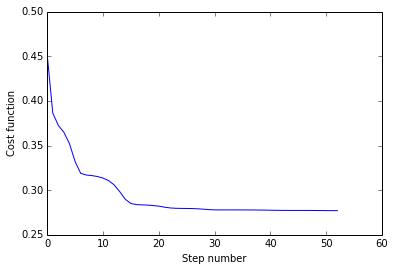## Neural Network¶

Neural Networks allow to construct non-linear models by connecting several layers of linear units, typically logistic regression, which results in a final output that can capture non-linear dependencies between the variable. If the dependency is mostly linear, there won't be much advantage in using a Neural Network though, as it seems to be the case with this example (compare the success rate between the LR and the NN algoriths.)

In :
# Parameters for the NN algorithm, run this cell before running the predictor!

filename = "learn-data.csv"

L = 1             # Number of hidden layers
hf = 1            # Factor to calculate number of hidden units given the number of variables
gamma = 0.002     # Regularization coefficient

threshold = 1E-5  # Default convergence threshold

showp = True      # Show minimization plot
gcheck = False    # Gradient check
testMode = False  # Test mode: runs the algorithm testCount times
testCount = 1     # Number of test runs

In :
# This code implements a neural network predictor. The dependent variable
# must be binary and the first column in the data frame, and all the independent
# categorical variables must be binary as well.
# Requires:
# pandas http://pandas.pydata.org/
# numpy http://www.numpy.org/

import sys
import math
import pandas as pd
import numpy as np
from scipy.optimize import fmin_bfgs
import matplotlib.pyplot as plt

def thetaMatrix(theta, N, L, S, K):
# The cost argument is a 1D-array that needs to be reshaped into the
# parameter matrix for each layer:
thetam = [None] * L
C = (S - 1) * N
thetam = theta[0 : C].reshape((S - 1, N))
for l in range(1, L - 1):
thetam[l] = theta[C : C + (S - 1) * S].reshape((S - 1, S))
C = C + (S - 1) * S
thetam[L - 1] = theta[C : C + K * S].reshape((K, S))
return thetam

def gradientArray(gmatrix, N, L, S, K):
garray = np.zeros((S - 1) * N + (L - 2) * (S - 1) * S + K * S)
C0 = (S - 1) * N
# http://docs.scipy.org/doc/numpy/reference/generated/numpy.copyto.html
np.copyto(garray[0 : C0], gmatrix.reshape(C0))
C = C0
for l in range(1, L - 1):
Ch = (S - 1) * S
np.copyto(garray[C : C + Ch], gmatrix[l].reshape(Ch))
C = C + Ch
Ck =  K * S
np.copyto(garray[C : C + Ck], gmatrix[L - 1].reshape(Ck))

return garray

def sigmoid(v):
return 1 / (1 + np.exp(-v))

def forwardProp(x, thetam, L):
a = [None] * (L + 1)
a = x
for l in range(0, L):
z = np.dot(thetam[l], a[l])
res = sigmoid(z)
a[l + 1] = np.insert(res, 0, 1) if l < L - 1 else res
return a

def backwardProp(y, a, thetam, L, N):
err = [None] * (L + 1)
err[L] = a[L] - y
for l in range(L - 1, 0, -1):
backp = np.dot(np.transpose(thetam[l]), err[l + 1])
deriv = np.multiply(a[l], 1 - a[l])
err[l] = np.delete(np.multiply(backp, deriv), 0)
err = np.zeros(N);
return err

def cost(theta, X, y, N, L, S, K, gamma):
M = X.shape

# The cost argument is a 1D-array that needs to be reshaped into the
# parameter matrix for each layer:
thetam = thetaMatrix(theta, N, L, S, K)

h = np.zeros(M)
terms = np.zeros(M)
for i in range(0, M):
a = forwardProp(X[i,:], thetam, L)
h[i] = a[L]
t0 = -y[i] * np.log(h[i]) if 0 < y[i] else 0
t1 = -(1-y[i]) * np.log(1-h[i]) if y[i] < 1 else 0
if math.isnan(t0) or math.isnan(t1):
#print "NaN detected when calculating cost contribution of observation",i
terms[i] = 10
else:
terms[i] = t0 + t1

# Regularization penalty
penalty = (gamma/2) * np.sum(theta * theta)

return terms.mean() + penalty;

def gradient(theta, X, y, N, L, S, K, gamma):
M = X.shape

# The cost argument is a 1D-array that needs to be reshaped into the
# parameter matrix for each layer:
thetam = thetaMatrix(theta, N, L, S, K)

# Init auxiliary data structures
delta = [None] * L
D = [None] * L
delta = np.zeros((S - 1, N))
D = np.zeros((S - 1, N))
for l in range(1, L - 1):
delta[l] = np.zeros((S - 1, S))
D[l] = np.zeros((S - 1, S))
delta[L - 1] = np.zeros((K, S))
D[L - 1] = np.zeros((K, S))

for i in range(0, M):
a = forwardProp(X[i,:], thetam, L)
err = backwardProp(y[i], a, thetam, L, N)
for l in range(0, L):
# Notes about multiplying numpy arrays: err[l+1] is a 1-dimensional
# array so it needs to be made into a 2D array by putting inside [],
# and then transposed so it becomes a column vector.
prod = np.array([err[l+1]]).T * np.array([a[l]])
delta[l] = delta[l] + prod

for l in range(0, L):
D[l] = (1.0 / M) * delta[l] + gamma * thetam[l]

grad = gradientArray(D, N, L, S, K)

global gcheck
if gcheck:
ok = True
size = theta.shape
epsilon = 1E-5
maxerr = 0.01
grad1 = np.zeros(size);
for i in range(0, size):
theta0 = np.copy(theta)
theta1 = np.copy(theta)

theta0[i] = theta0[i] - epsilon
theta1[i] = theta1[i] + epsilon

c0 = cost(theta0, X, y, N, L, S, K, gamma)
c1 = cost(theta1, X, y, N, L, S, K, gamma)
grad1[i] = (c1 - c0) / (2 * epsilon)
diff = abs(grad1[i] - grad[i])
if maxerr < diff:
print "Numerical and analytical gradients differ by",diff,"at argument",i,"/",size
ok = False
if ok:
print "Numerical and analytical gradients coincide within the given precision of",maxerr

return grad

def predict(x, theta, N, L, S, K):
thetam = thetaMatrix(theta, N, L, S, K)
a = forwardProp(x, thetam, L)
h = a[L]
return h;

def debug(theta):
global params
global values
(X, y, N, L, S, K, gamma) = params
value = cost(theta, X, y, N, L, S, K, gamma);
values = np.append(values, [value])
#print value

# Main -----------------------------------------------------------------------------

K = 1

if L < 1:
print "Need to have at least one hidden layer"
sys.exit(1)

L = L + 1

# Loading data frame and initalizing dimensions
df = pd.read_csv(filename, delimiter=',', na_values="\\N")
M = df.shape
N = df.shape
S = int((N - 1) * hf) # add 1 for the bias unit on each layer
print 'Number of data samples          :', M
print 'Number of independent variables :', N-1
print 'Number of hidden layers         :', L-1
print 'Number of units per hidden layer:', S-1
print 'Number of output classes        :', K

y = df.values[:,0]

# Building the (normalized) design matrix
X = np.ones((M, N))
for j in range(1, N):
# Computing i-th column. The pandas dataframe
# contains all the values as numpy arrays that
# can be handled individually:
values = df.values[:, j]
minv = values.min()
maxv = values.max()
X[:, j] = (values - minv) / (maxv - minv)

rates = np.array([])
for iter in range(0, testCount):
if testMode: print "-------> Iteration test",iter

# Create training set by randomly choosing 70% of rows from each output
# category
i0 = np.where(y == 0)
i1 = np.where(y == 1)
ri0 = np.random.choice(i0, size=0.7*i0.shape, replace=False)
ri1 = np.random.choice(i1, size=0.7*i1.shape, replace=False)
itrain = np.concatenate((ri1, ri0))
itrain.sort()

Xtrain = X[itrain,:]
ytrain = y[itrain]

# Number of parameters:
# * (S - 1) x N for the first weight natrix (N input nodes counting the bias term), into S-1 nodes in
#   the first hidden layer
# * (S - 1) x S for all the weight matrices in the hidden layers, which go from S (counting bias term)
#   into S-1 nodes in the next layer. Since L counts th number of hidden layers plus the output layer,
#   and the first transition was accounted by the first term, then we only need L-2
# * K x S, for the last transition into the output layer with K nodes
theta0 = np.random.rand((S - 1) * N + (L - 2) * (S - 1) * S + K * S)
params = (Xtrain, ytrain, N, L, S, K, gamma)

# http://docs.scipy.org/doc/scipy/reference/generated/scipy.optimize.fmin_bfgs.html
print "Training Neural Network..."
values = np.array([])
thetaOpt = fmin_bfgs(cost, theta0, fprime=gradient, args=params, gtol=threshold, callback=debug)
print "Done!"

# Calculating the prediction rate by applying the trained model on the remaining
# 30% of the data (the test set), and comparing with random selection
ntot = 0
nhit = 0
nran = 0
for i in range(0, M):
if not i in itrain:
ntot = ntot + 1
p = predict(X[i,:], thetaOpt, N, L, S, K)
if (y[i] == 1) == (0.5 < p):
nhit = nhit + 1
r = np.random.rand()
if (y[i] == 1) == (0.5 < r):
nran = nran + 1

rate = float(nhit) / float(ntot)
rrate = float(nran) / float(ntot)
rates = np.append(rates, [rate])

print ''
print '---------------------------------------'
print 'Predictor success rate on test set:', round(100 * rate, 2), '%'
print 'Random success rate on test set   :', round(100 * rrate, 2), '%'

if showp and not testMode:
plt.plot(np.arange(values.shape), values)
plt.show()

if testMode:
print ''
print '***************************************'
print 'Average success rate:', round(100 * np.average(rates), 2), '%'
print 'Standard deviation  :', round(100 * np.std(rates, ddof=1), 2), '%'

Number of data samples          : 155
Number of independent variables : 9
Number of hidden layers         : 1
Number of units per hidden layer: 8
Number of output classes        : 1
Training Neural Network...
Optimization terminated successfully.
Current function value: 0.331249
Iterations: 404
Function evaluations: 411
Gradient evaluations: 411
Done!

---------------------------------------
Predictor success rate on test set: 85.11 %
Random success rate on test set   : 55.32 %